Courses
Courses for Kids
Free study material
Offline Centres
More

# Where to Look from Class 3 Notes CBSE Maths Chapter 1 (Free PDF Download)Last updated date: 02nd Dec 2023
Total views: 164.1k
Views today: 2.64k

## NCERT Class 3 Maths Chapter 1 Where to Look From Revision Notes

The idea of shapes and angles of 2D and 3D objects has been introduced in Class 3 Maths Chapter 1 Where to Look From. So in this chapter, students will learn the concepts of geometric shapes and angles and will find out how an object looks from a particular angle.

Download and refer to the Where to Look From revision notes to understand the concepts of basic geometry easily. These notes will help the young students of Class 3 to comprehend the ideas of how an object looks from different angles. They will learn how to identify and draw patterns by following the explanation of the subject experts.

Also, check CBSE Class 3 Maths revision notes for other chapters:

## Revision Notes Class 3 Mathematics

### Introduction to Different Kinds of Views

• Side View:  When a person views an object from the side instead of front or back.

• Front View: When an object is viewed from the front by an observer.

• Back View:  When an object is viewed from the back by an observer.

• Top View: When an object is viewed from the top by an observer.

• Bottom View: When an object is viewed from the bottom by an observer.

### Solved Examples

1. Which of the following figures has the top view of a table?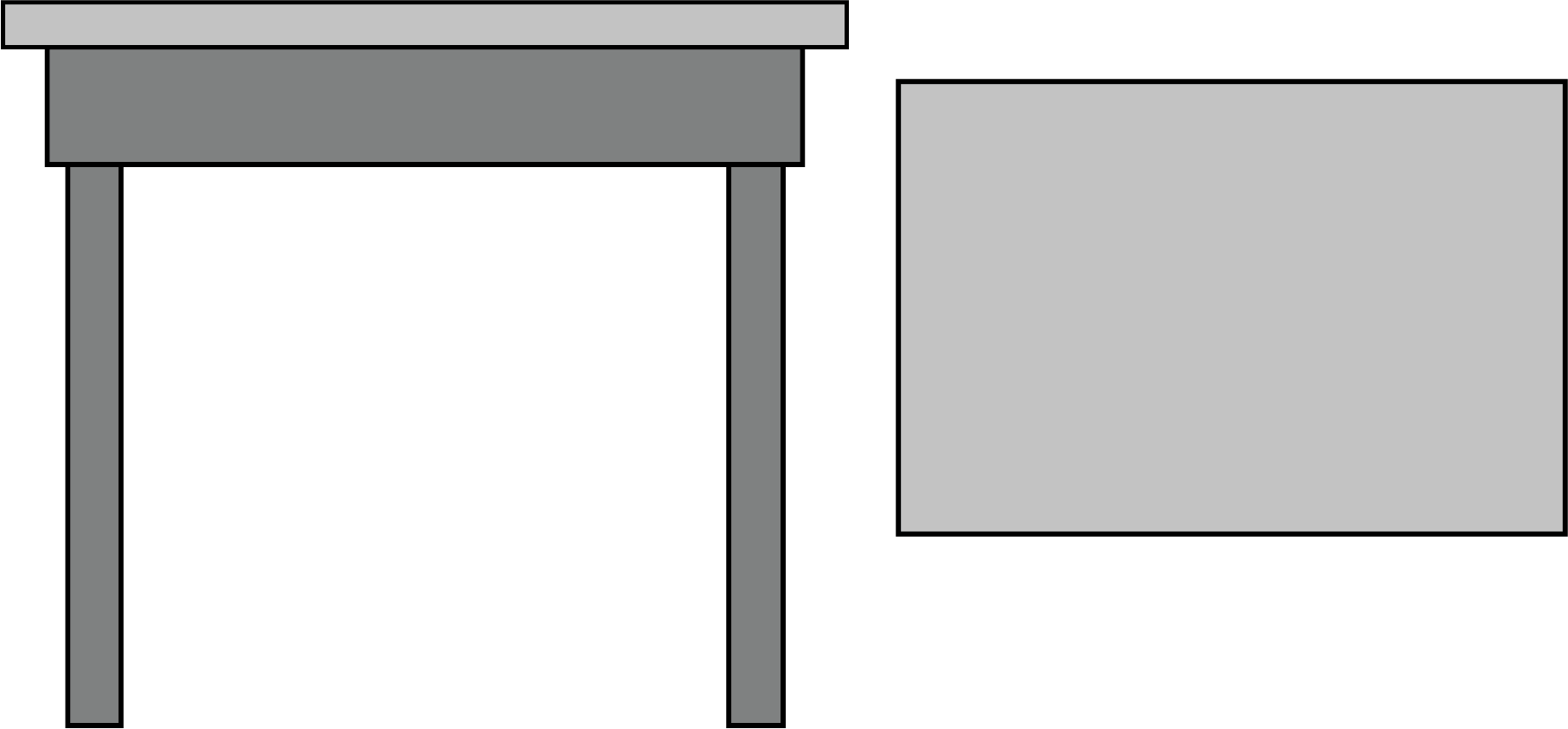Side and Top View of The table

Ans. From the above two figures, the First figure shows a side view of a  table, when we will observe the table from the top, the top view of the table will be the below-given figure

2. Draw the side, front and top view of the following given figure?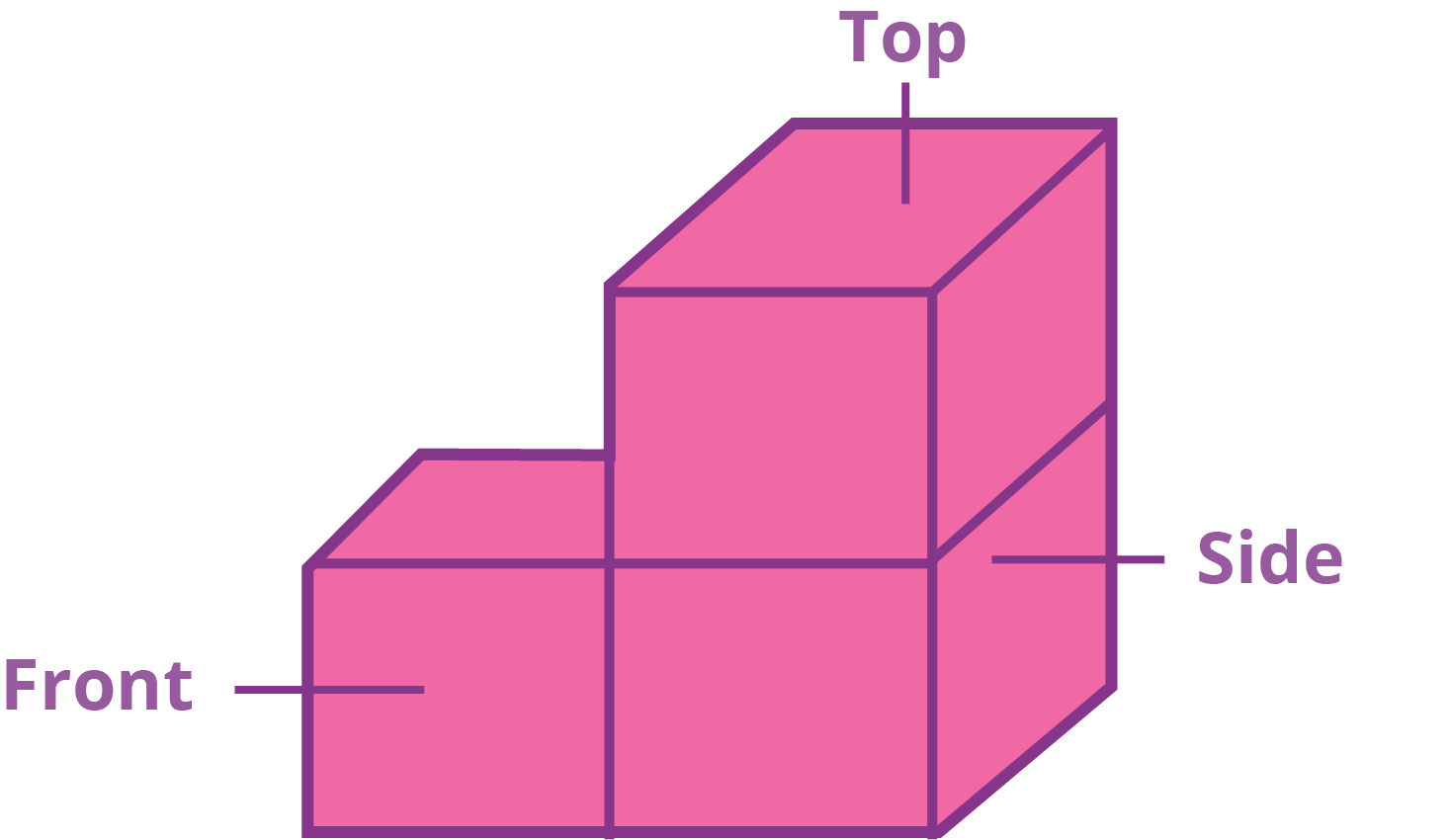Views of Squares Blocks

Ans. The  side, front and  top views of the above figure are given below :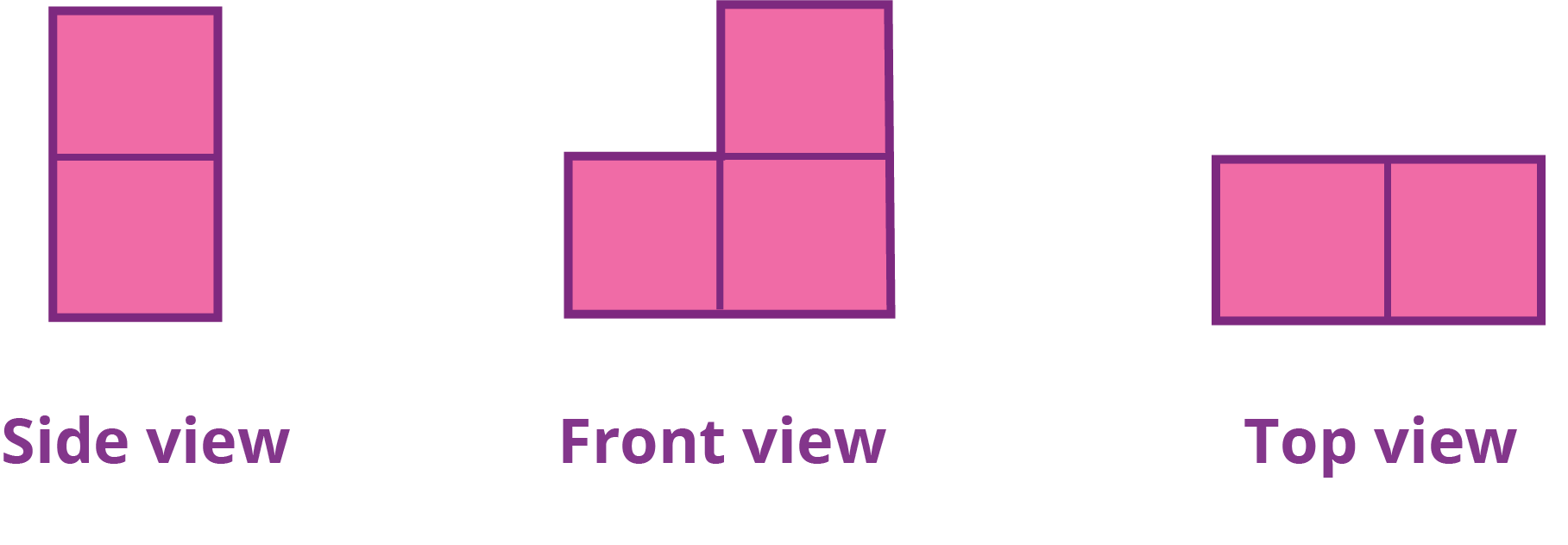Side, Front and Top View of Square Blocks

• In the side view of a figure, we will observe two square blocks.

• In the front view of a figure, we will observe three square blocks.

• In the top view of a figure, we will observe two square blocks.

3. Draw the front, side and top view of the given figure?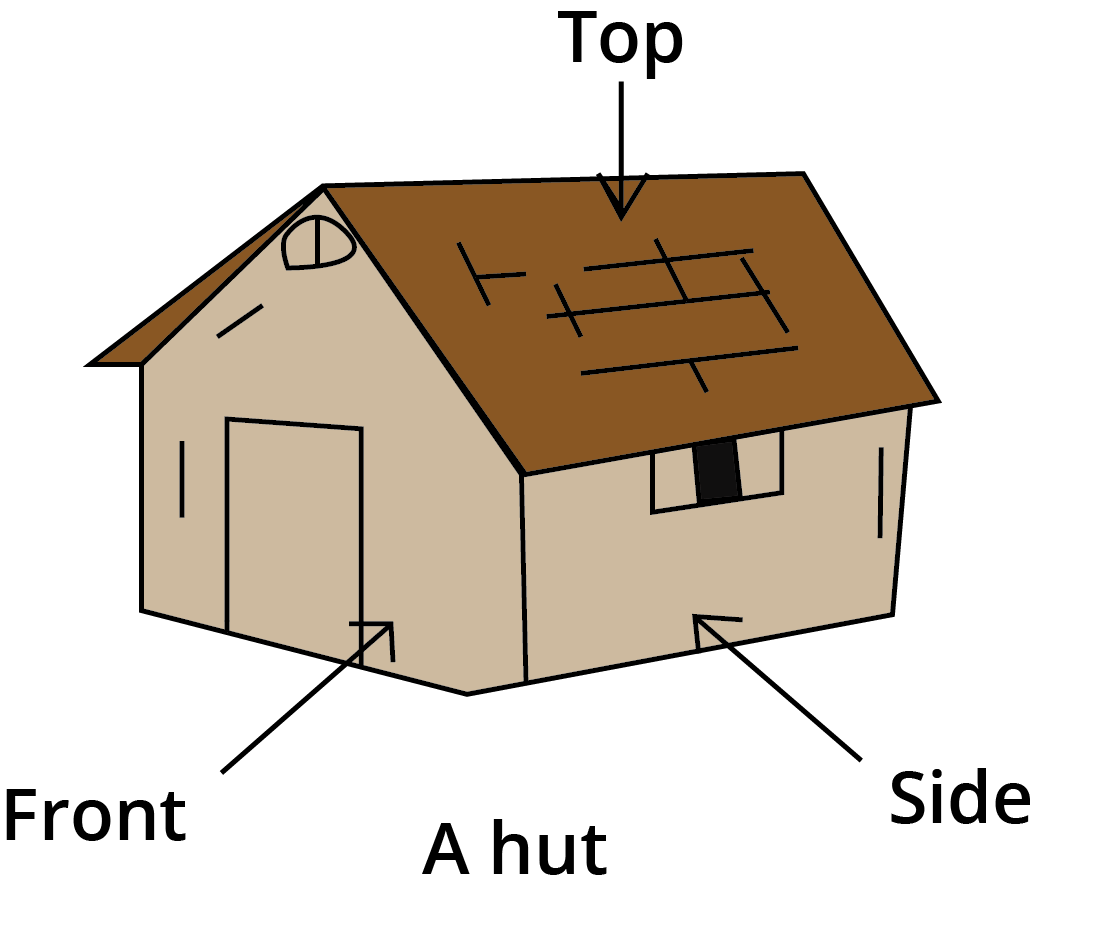Different View of The Hut

Ans. The top, front and side views are shown below :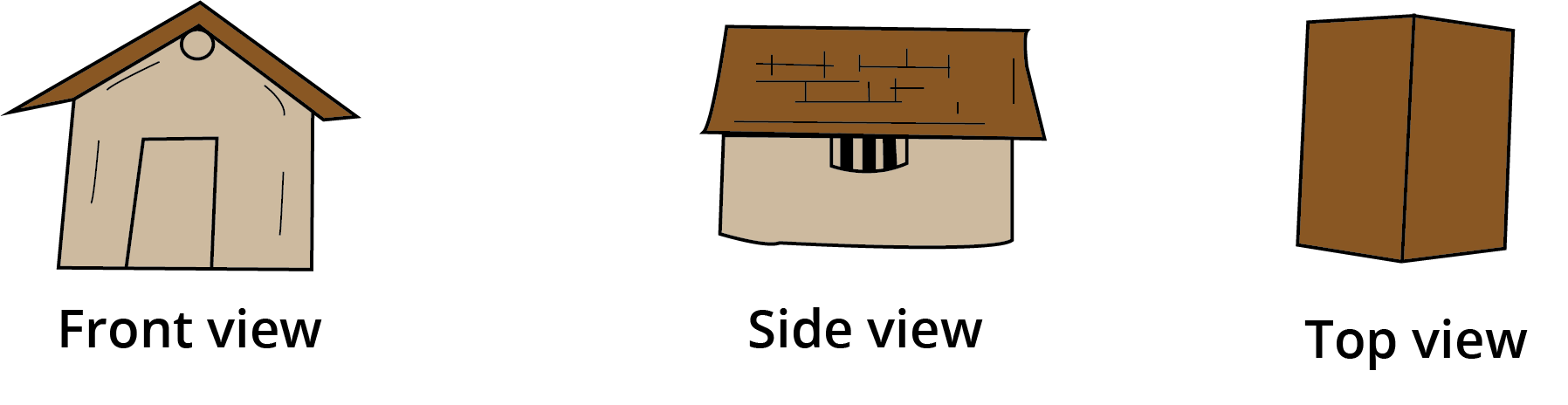Front View, Side View and Top View of a Hut

### Rangoli Patterns

• We use Rangoli Patterns in Maths to find symmetry and reflection.

• It can be done by joining the dotted lines on the dot grid to find symmetry and the reflection of different figures.

• In mathematics,  the rangoli symbols go on expanding to form a line and the basic geometrical shapes like the circle, triangle, square, rectangle and so on

### Solved Example

1. A dot grid is given below, you are required to draw a kite, leaf, flower, boat, star and a pot on it?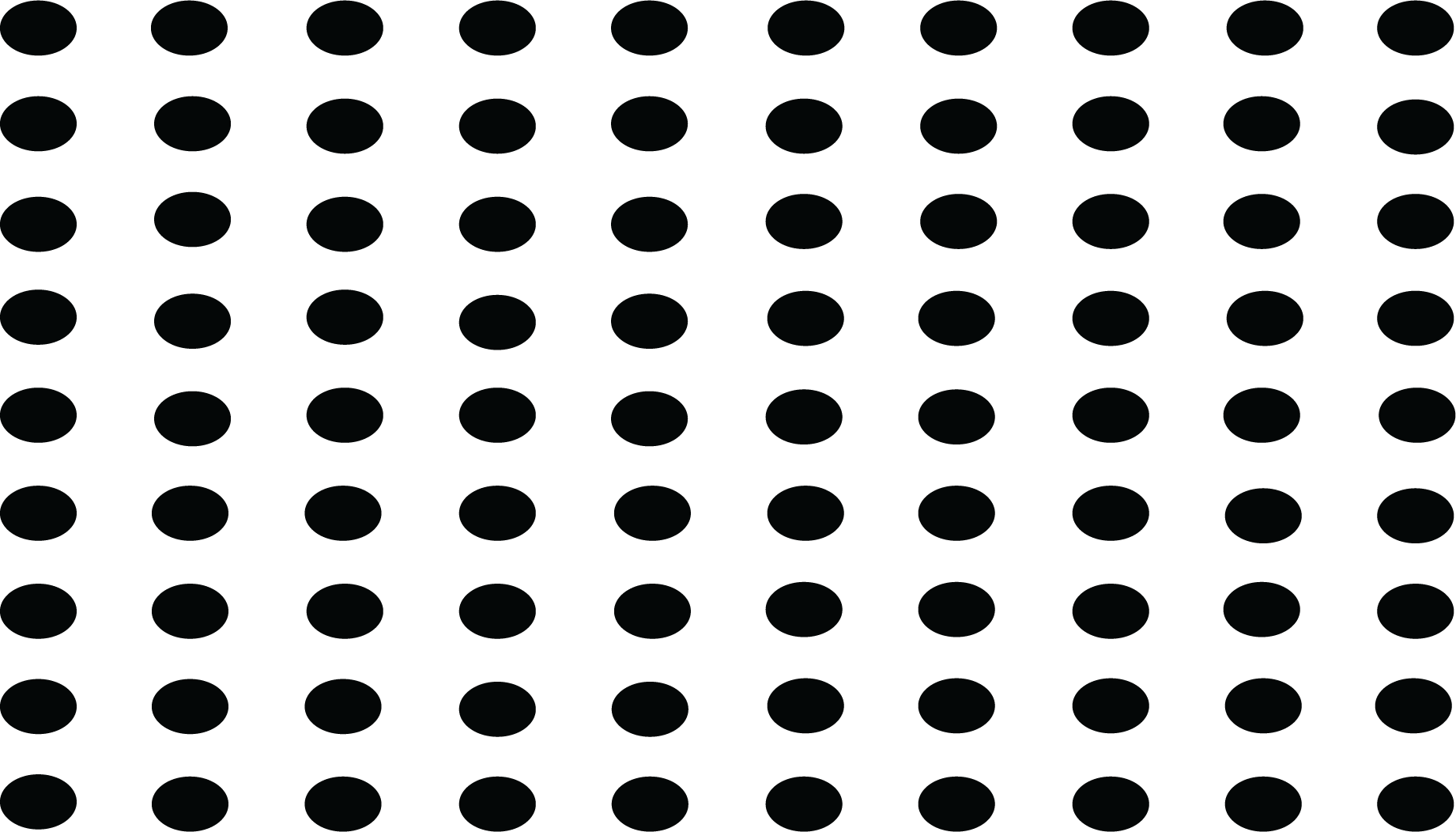Dot Grid

Ans: The required figures on the dot grid are drawn given below :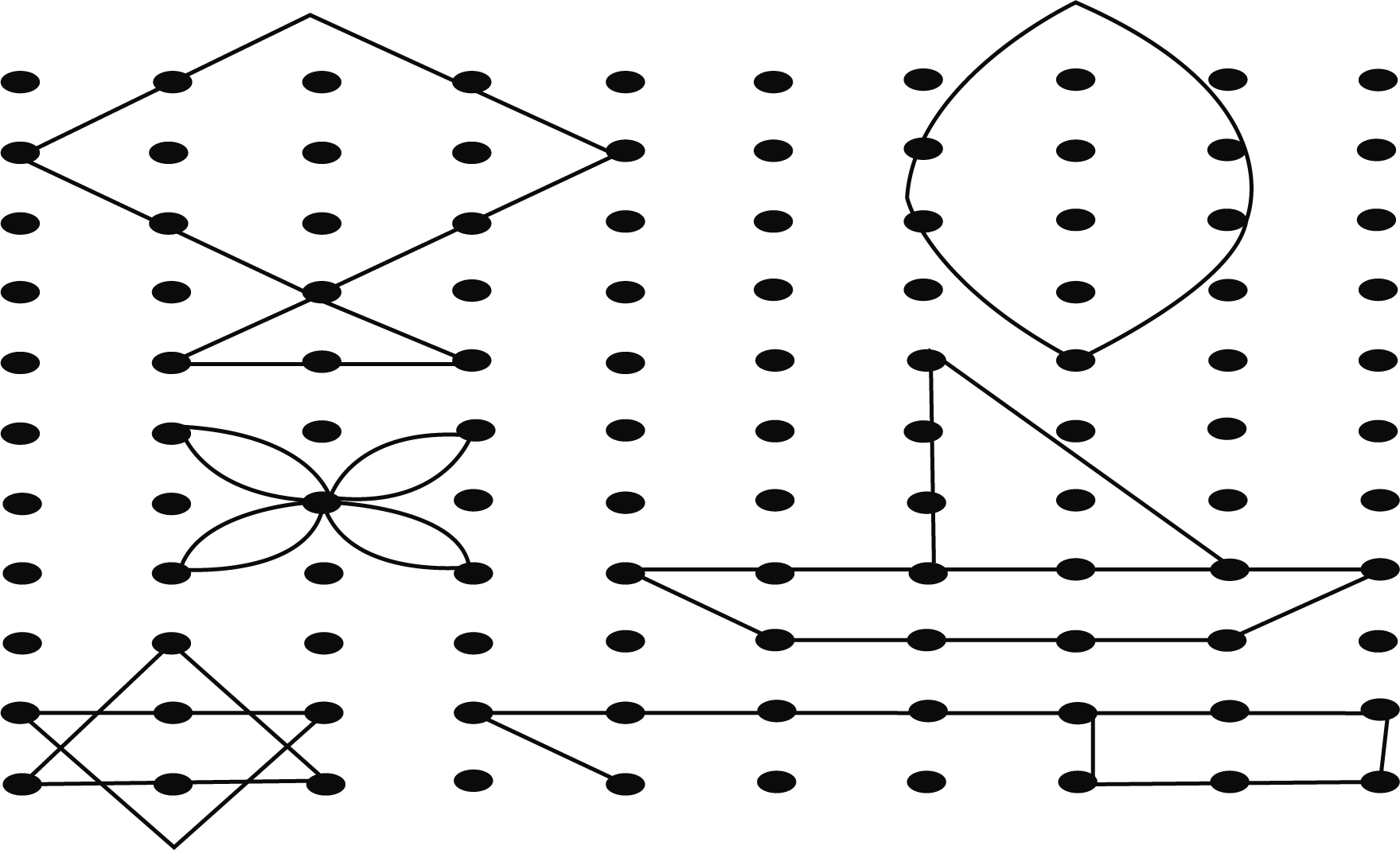Shape Formation by Dot Grid

We can observe a kite, leaf, flower, boat, star and pot in the above figure.

### Mirror Halves

• Mirror ( or reflection ) symmetry divides a figure or design into halves that are mirror images.

• In other words, objects are the same on both sides of a line ( usually in the middle).

• Example of Mirror Halves are wings of Butterfly can be exactly get halved and Letter words like H, Y W X are the examples of Mirror halves as they can be exactly cut into two halves

### Solved Example

1. Draw the Mirror halves of the following figures?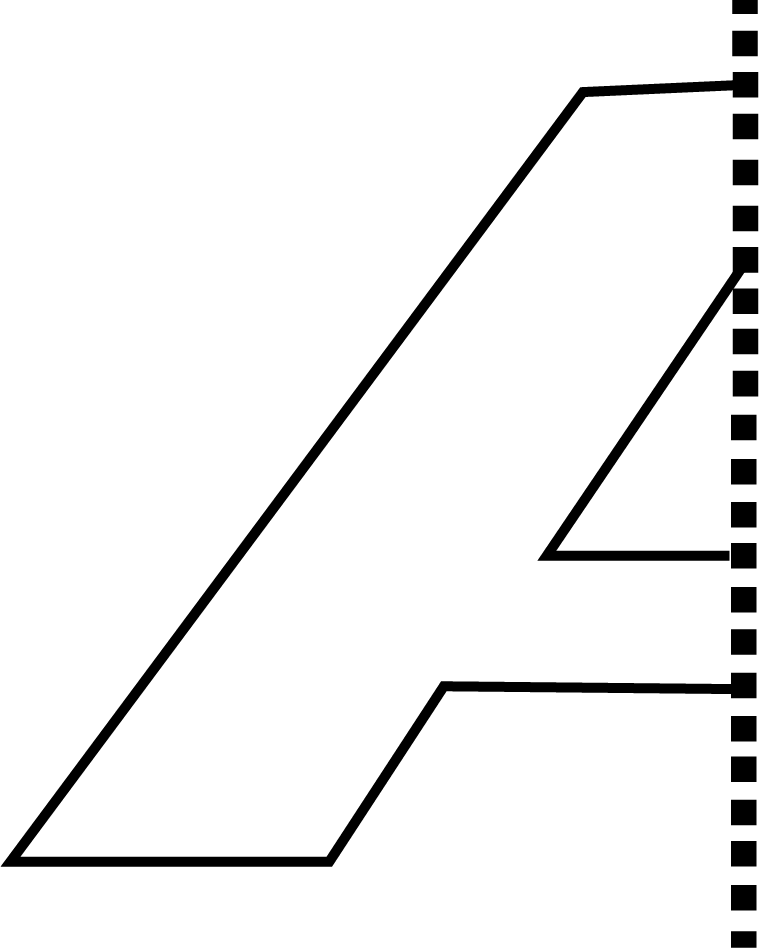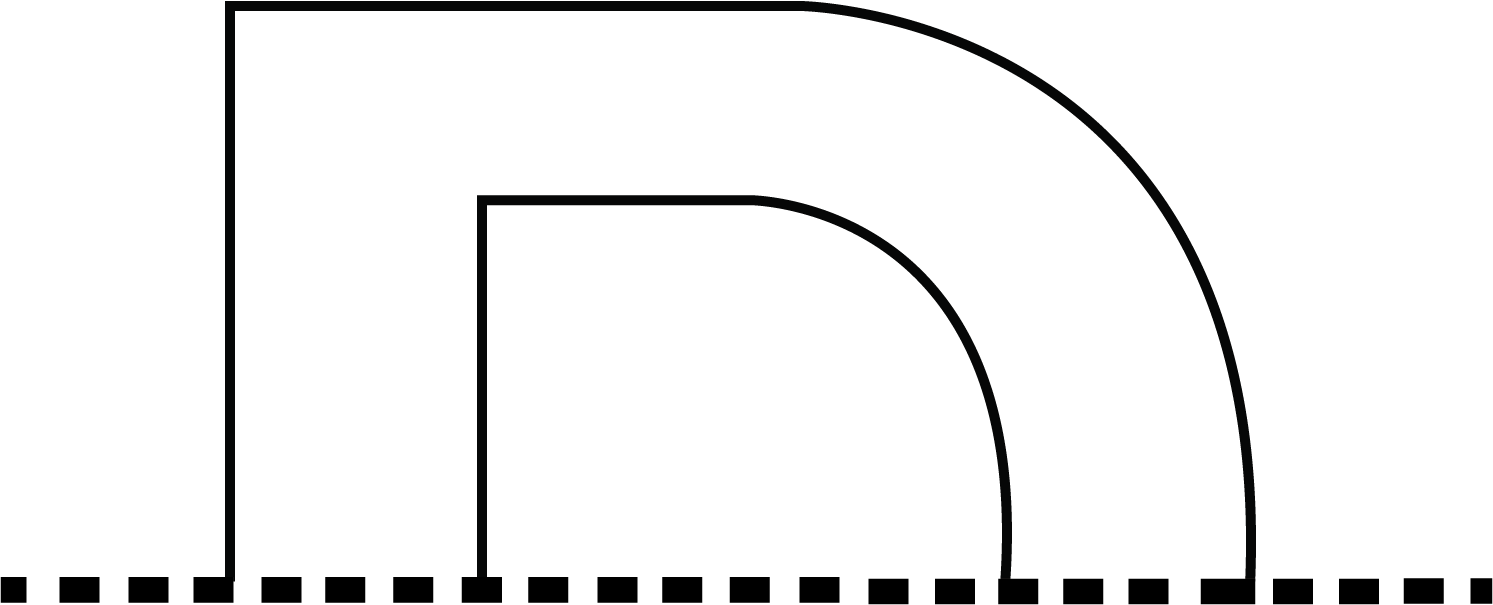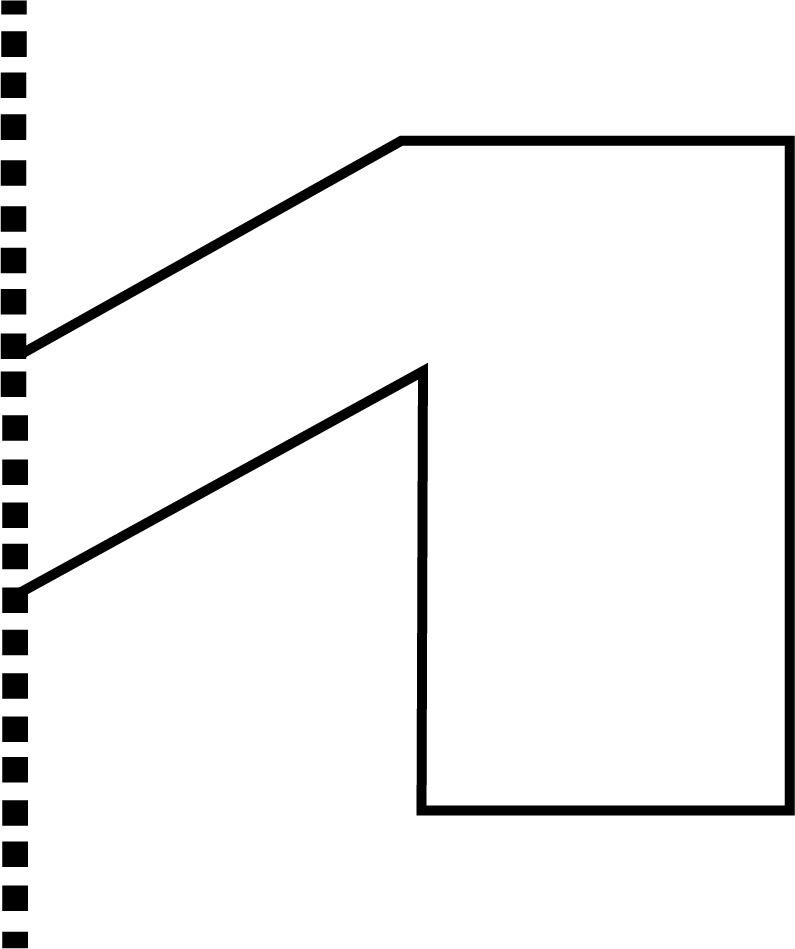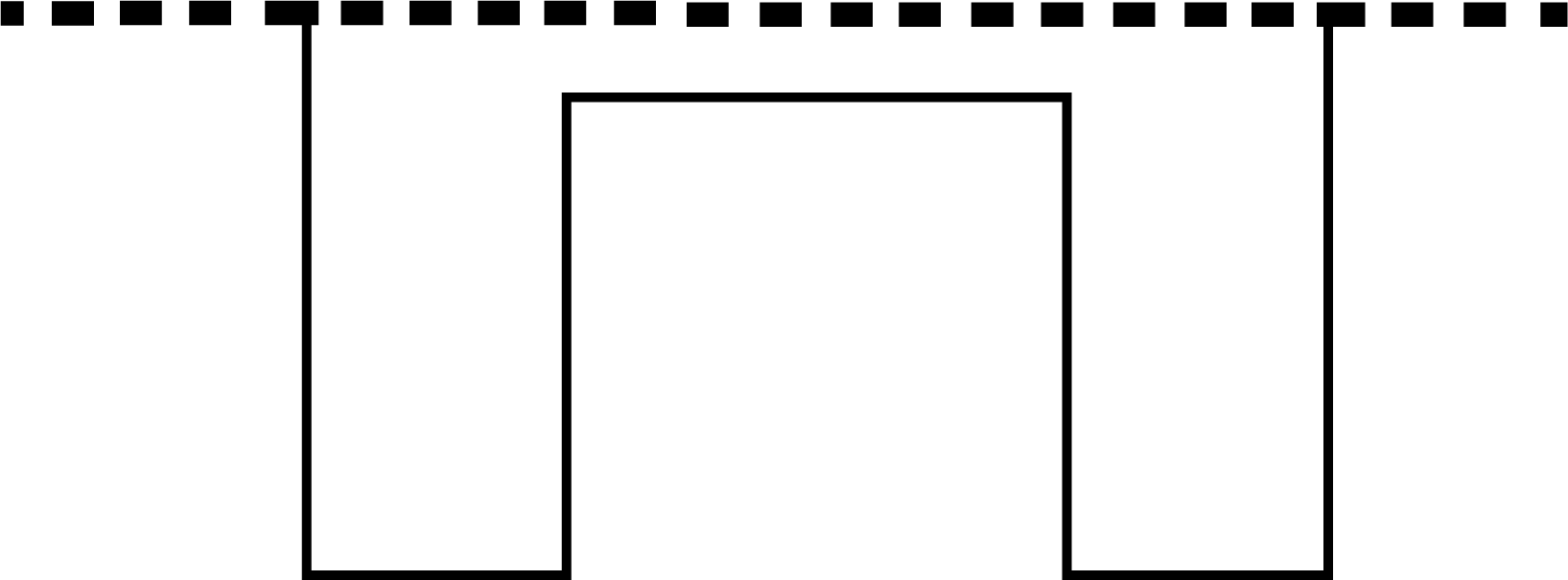Mirror Halves

Ans.

• Figure(a) clearly shows the Mirror half of the capital letter A, and we have to draw the other mirror half of it. The required other mirror half is given below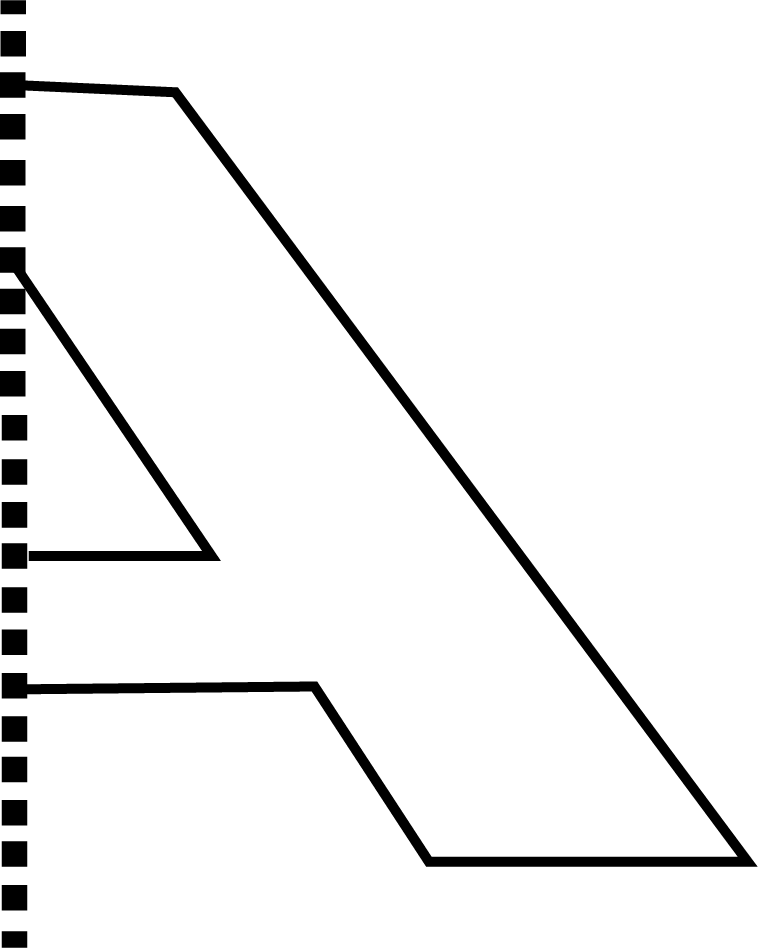Mirror Image of Half A

• Figure(b) clearly shows the Mirror half of the capital letter D, and we have to draw the other mirror half of it. The required other mirror half is given below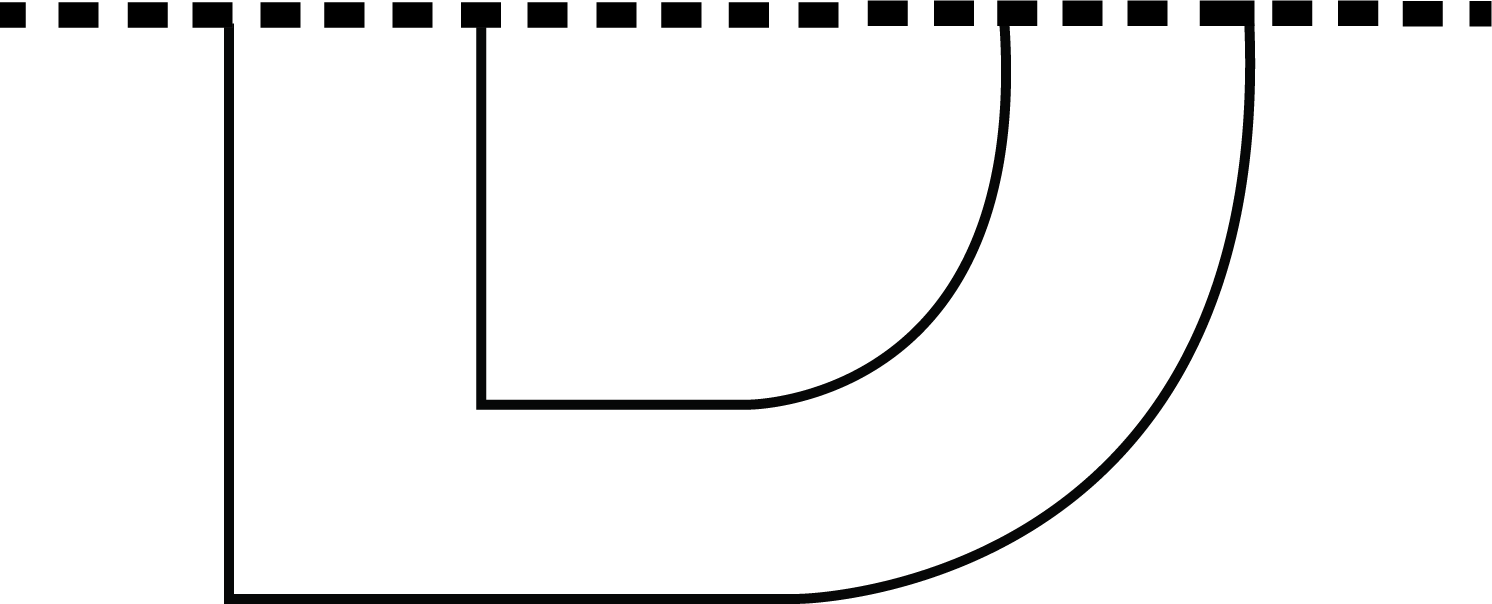Mirror Image of Half D

• Figure(c) clearly shows the Mirror half of the capital letter N, and we have to draw the other mirror half of it. The required other mirror half is given belowMirror Image of Half N

• Figure(d) clearly shows the Mirror half of the capital letter H, and we have to draw the other mirror half of it. The required other mirror half is given below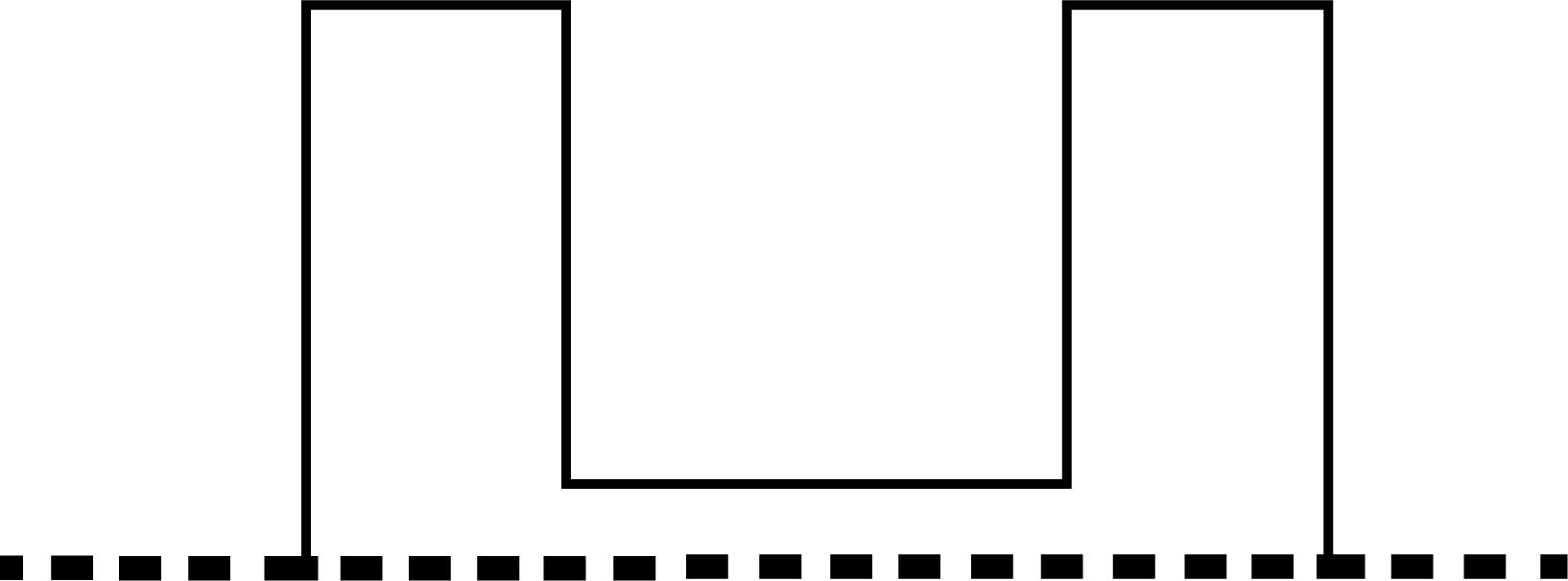Mirror Image of Half H

### Reflection Symmetry

• When the first half of an object reflects the other half of the object in the mirror, then we call it Reflection Symmetry.

• Reflection Symmetry can also be named Mirror Symmetry.

• For example, in general, human faces are identical on the left and right sides. The wings of most butterflies are identical on both sides, the left and right sides.

### Finding Reflection Symmetry

• The first half of an object must be a reflection of the other half of an object.

• Imagine folding a rectangle along each line of symmetry and each of the half matching up perfectly, this is symmetry

• For the two symmetrical halves, one of them follows lateral inversion, that is the left side appears to be the right side as it happens when you look in a mirror.

• For Example The reflection of trees in clear water, and the reflection of mountains in a lake are among the commonly seen examples of reflection symmetry around us.

### Practise Problems

Q1. Draw some figures out of your imagination which can be exactly cut into two halves?

Q2. Below are some given pictures, and you have to think from what kind of view these pictures will seem like this?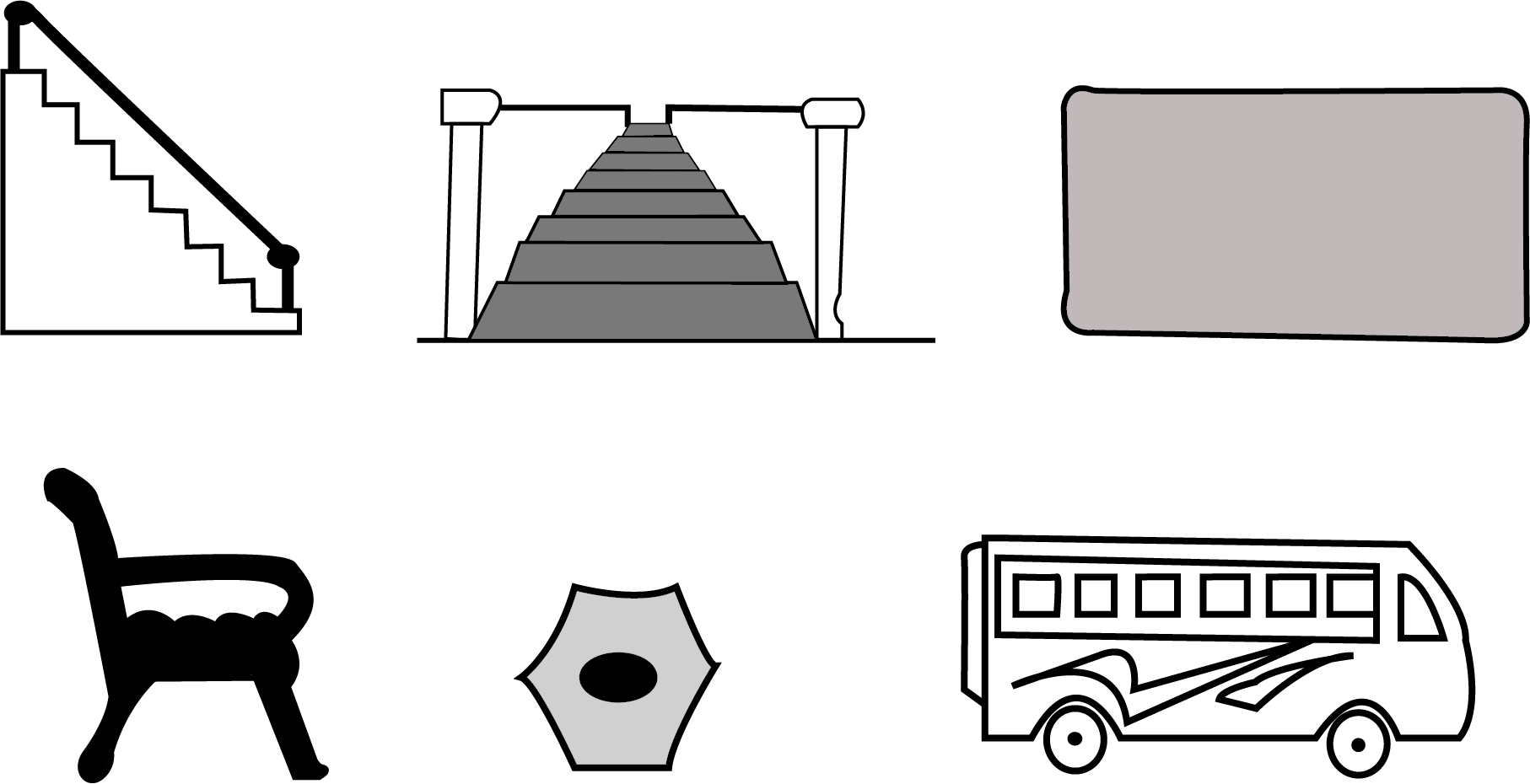Different Kinds of Views

Ans: Below is some required figures that can be exactly cut into two halves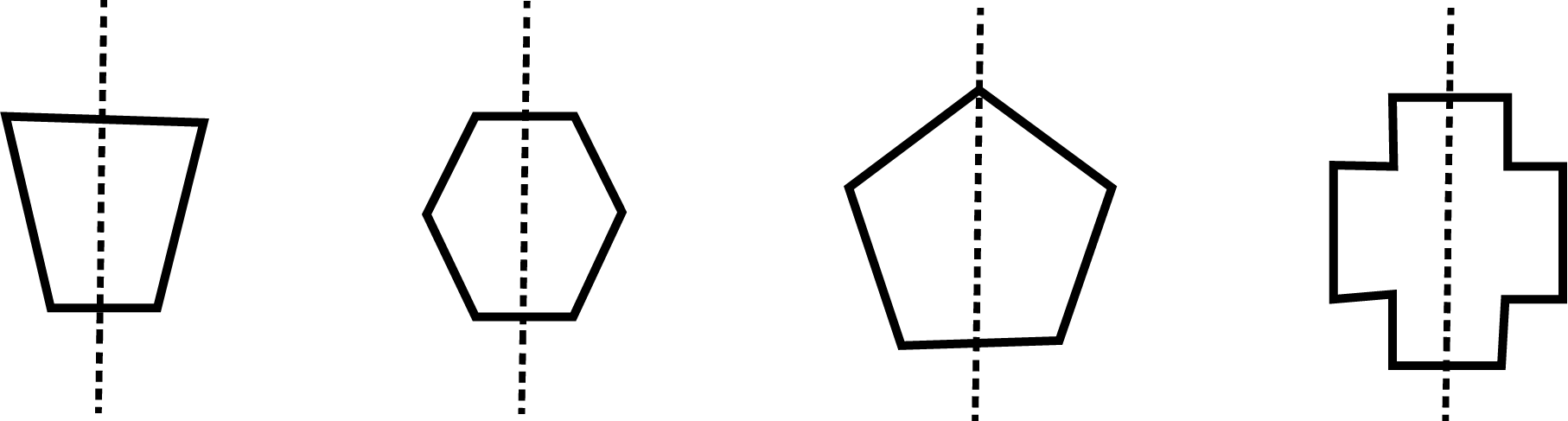Equal Halves

Ans:

• Staircase - Side View is seen

• Staircase - Top View is seen

• Table - Top View is seen

• Chair - Side View is seen

• Pencil - Top View is seen

• Bus - Side View is seen

## Importance of Where to Look From Class 3 Maths Worksheets

This chapter has been formulated with the prime aim of instilling skills to recognize the different sections of images and objects. It helps the students of Class 3 to identify the mirror halves of alphabets and images. They will also gain an understanding of what images look like in a reflection, with the help of the Where to Look From Class 3 Maths worksheets.

This chapter is important in terms of creating a better understanding of geometrical figures. These revision notes will encourage students of Class 3 to think of the actual representation of images and objects we see every day.

This perception of shapes and level of visibility of objects from 2D images will develop new skills related to 2D and 3D geometry. Class 3 students will understand what part of the objects will be visible if looked at from a particular angle.

Proceeding to the next level of this chapter, students will learn what the mirror halves of objects and images look like. They will get to learn what lateral inversion is by studying the mirror halves of images, alphabets, and objects. Studying a mirror half image will develop exceptional skills to identify, draw, and comprehend shapes for higher classes.

## Benefits of Where to Look From Class 3 Maths Worksheets

Apart from the exercise questions, the young inquisitive minds will need something to quench their zeal. So we have provided well-organized worksheets for their practice. They can download the Where to Look From Class 3 Maths PDF to get the worksheets of this chapter, prepared by our subject matter experts.

The questions in the worksheets are framed with the prime objective to check the level of understanding of the concepts among students. These worksheet questions will also have answers. After having finished studying Where to Look From, students can focus on solving the worksheets.

Solving a worksheet will help them evaluate their skills to perceive geometrical images and their specific angles. Students will also be able to comprehend 2D and 3D geometry easily in higher classes.

So enjoy studying the concise and accurate Where to Look From revision notes and use them as references when you are preparing for the exams. You can also refer to the other relevant study materials for this chapter on Vedantu.

## FAQs on Where to Look from Class 3 Notes CBSE Maths Chapter 1 (Free PDF Download)

1. Is the Class 3 Maths Chapter 1 revision notes free to download?

The revision notes for this chapter are free to download. You can get it in PDF format on Vedantu mobile app and official website.

2. What will you learn from the revision notes of Class 3 Maths Chapter 1?

You will learn how an object looks from a particular angle in the revision notes of Class 3 Maths Chapter 1. Study the concepts and understand what the mirror halves of alphabets and objects look like from the revision notes of this chapter.

3. Where can I get NCERT Solutions for Class 3 Maths Chapter 1?

You can get NCERT Solution for Class 3 Maths Where to Look From at Vedantu. All the study materials are available for free download.